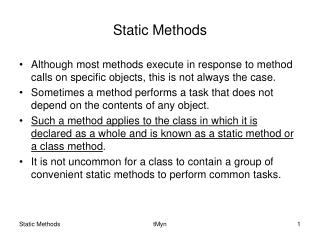DownloadDownload PresentationStatic Methods

Static Methods

Télécharger la présentationStatic Methods

- - - - - - - - - - - - - - - - - - - - - - - - - - - E N D - - - - - - - - - - - - - - - - - - - - - - - - - - -
Presentation Transcript

1. Static Methods • Although most methods execute in response to method calls on specific objects, this is not always the case. • Sometimes a method performs a task that does not depend on the contents of any object. • Such a method applies to the class in which it is declared as a whole and is known as a static method or a class method. • It is not uncommon for a class to contain a group of convenient static methods to perform common tasks. tMyn

2. To declare a method as static, place the keyword static before the return type in the method’s declaration. • You can call any static method by specifying the name of the class in which the method is declared, followed by a dot (.) and the method name: ClassName.methodName(arguments); • For example the class Math provides a collection of static methods that enable you to perform common mathematical calculations. tMyn

3. package staticmethod; public class Main { public static void main(String[] args) { System.out.println("The square root of 6789.932 is "+ Math.sqrt(6789.932)); } } run: The square root of 6789.932 is 82.40104368271072 BUILD SUCCESSFUL (total time: 1 second) tMyn

4. Note that there was no need to create a Math object before calling method public static double sqrt(double a). • Also, note that all Math class methods are static – therefore, each is called by preceding the name of the method with the class name Math and a dot separator. tMyn

5. Next example uses static method static int getCount() to count the number of objects created in the class. Here we also use one static field, count. • A given class will have only one copy of each of its static fields or class variables, and these will be shared between and among all the objects of the class. • Each class variable exists even if no objects of the class have been created. • Class variables belong to the class, and they can be referenced by any object or class method, not just methods belonging to instances of that class. tMyn

6. If the value of a static field is changed, the new value is available equally in all the objects of the class. • This is quite different from non-static fields, where changing a value for one object does not affect the values in another objects. • A static field must be declared using keyword static preceding the type name. tMyn

7. package TimoSoft; public class Rectangle { double width, height; static int count=0; Rectangle(double wVal, double hVal) { setWidth(wVal); setHeight(hVal); ++count; } void setWidth(double w) { width=w; } tMyn

8. void setHeight(double h) { height=h; } void getWidth() { System.out.println("The width is "+width); } void getHeight() { System.out.println("The height is "+height); } static int getCount() { return count; } } tMyn

9. package TimoSoft; public class RectangleTest { public static void main(String[] args) { Rectangle first=new Rectangle(12.5, 7.9); Rectangle second=new Rectangle(11.5, 4.8); Rectangle third=new Rectangle(33.6, 88.1); System.out.println("In the end there are "+Rectangle.getCount()+ " objects in the class."); } } run: In the end there are 3 objects in the class. BUILD SUCCESSFUL (total time: 5 seconds) tMyn